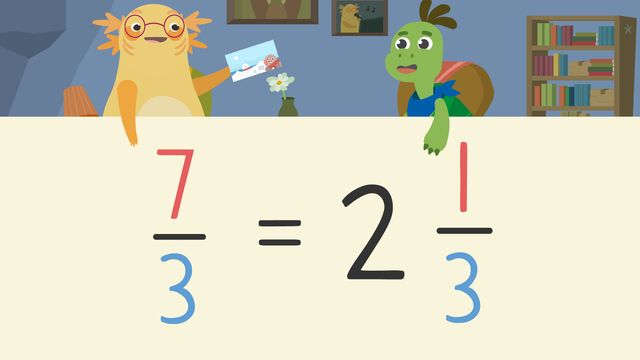# Improper Fractions and Mixed NumbersRate this video

Be the first to rate this video!

The authorTeam Digital
Improper Fractions and Mixed Numbers
CCSS.MATH.CONTENT.4.NF.B.3.C

## Information about the videoImproper Fractions and Mixed Numbers

### Contents

Axel and Tank received a postcard from their friend with a recipe for snow. However, there’s just one problem: they need to convert improper fractions to mixed numbers in order to see how much of each ingredient they need. Let’s read about this improper fractions to mixed numbers video so we can help Axel and Tank with converting improper fractions to mixed numbers.

### What is an improper fraction and What is a mixed number?

There are three types of fractions:

• proper fraction- the numerator is smaller than the denominator
• improper fraction- the numerator is larger than the denominator
• mixed number- a combination of a whole number and a fraction

Can you convert improper fractions to mixed numbers? The answer is yes, you can change improper fractions to mixed numbers. The next section will explain how to change improper fractions to mixed numbers using simple steps.

### Changing Improper Fractions to Mixed Numbers

How do you convert an improper fraction to a mixed number? We can convert, or change, improper fractions to mixed numbers so they are easier to understand. Here is an example of how to make improper fractions into mixed numbers:

• Step 1: Represent seven-thirds using fraction bars.• Step 2: Count how many wholes we have shaded in and write the whole number.• Step 3: Record the remaining part as a fraction.If you look closely at the image above you can see how to write improper fractions as mixed numbers. You will find that the answer is the mixed number is two and one third, once you follow the steps on how to turn improper fractions into mixed numbers.

### How to Convert Improper Fractions to Mixed Numbers Summary

How do you change improper fractions to mixed numbers? Remember, these steps explain how to turn improper fractions to mixed numbers:

• Step 1: Represent seven-thirds using fraction bars.
• Step 2: Count how many wholes we have shaded in and write the whole number.
• Step 3: Record the remaining part as a fraction.

Now, can you change improper fractions to mixed numbers? Practice how to convert improper fractions to mixed numbers on this website with the improper fractions to mixed numbers worksheet along with other activities, and exercises.